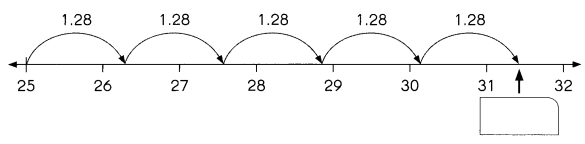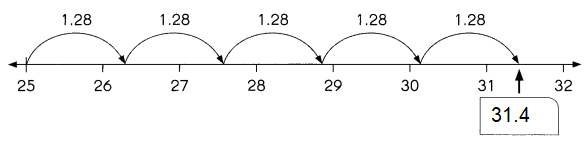# Math in Focus Grade 5 Chapter 9 Practice 1 Answer Key Multiplying Decimals

Practice the problems of Math in Focus Grade 5 Workbook Answer Key Chapter 9 Practice 1 Multiplying Decimals to score better marks in the exam.

## Math in Focus Grade 5 Chapter 9 Practice 1 Answer Key Multiplying Decimals

Multiply. Write the product as a decimal.

Example
2 × 0.3 = 2 × 3 tenths
= 6 tenths
0.6
So, 2 × 0.3 = 0.6

Question 1.
5 × 0.6 = 5 × ____ tenths
= ___ tenths
= ____ or ____
So, 5 × 0.6 = ____
5 × 0.6 = 5 × 6 tenths
= 30 tenths
= 3.0 or 3
So, 5 × 0.6 = 3
Explanation:
To multiply a decimal number by a decimal number,
we first multiply the two numbers ignoring the decimal points and then;
place the decimal point in the product in such a way that;
decimal places in the product is equal to the sum of the decimal places in the given numbers.

Question 2.
7 × 0.8 = 7 × ___ tenths
= ___ tenths
= _____
So, 7 × 0.8 = ___
7 × 0.8 = 7 × 8 tenths
= 56 tenths
= 5.6
So, 7 × 0.8 = 5.6
Explanation:
To multiply a decimal number by a decimal number,
we first multiply the two numbers ignoring the decimal points and then;
place the decimal point in the product in such a way that;
decimal places in the product is equal to the sum of the decimal places in the given numbers.

Question 3.
10 × 0.4 = 10 × ___ tenths
= ___ tenths or
= ___ or ___
So, 10 × 0.4 = ___
10 × 0.4 = 10 × 4 tenths
= 4 tenths or
= 4.0 or 4
So, 10 × 0.4 = 4
Explanation:
To multiply a decimal number by a decimal number,
we first multiply the two numbers ignoring the decimal points and then;
place the decimal point in the product in such a way that;
decimal places in the product is equal to the sum of the decimal places in the given numbers.

Multiply. Write the product as a decimal.

Example
3 × 0.03 = 3 × 3
= 9 hundredths
= 0.09
So, 3 × 0.03 = 0.09

Question 4.
5 × 0.02 = 5 × ___ hundredths
= ____ hundredths
= ___ or _____
So, 5 × 0.02 = ____
5 × 0.02 = 5 × 2 hundredths
= 10 hundredths
= 10/100 or 0.1
So, 5 × 0.02 = 0.1
Explanation:
To multiply decimals, first multiply as if there is no decimal.
Next, count the number of digits after the decimal in each factor.
Finally, when only one factor has decimal place value,
the product will have as many decimal place values as that one factor.

Question 5.
7 × 0.07 = 7 × ___ hundredths
= ____ hundredths
= ___ or _____
So, 7 × 0.07 = ____
7 × 0.07 = 7 × 7 hundredths
= 49 hundredths
=  49/100 or 0.49
So, 7 × 0.07 = 0.49
Explanation:
To multiply decimals, first multiply as if there is no decimal.
Next, count the number of digits after the decimal in each factor.
Finally, when only one factor has decimal place value,
the product will have as many decimal place values as that one factor.

Question 6.
6 × 0.12 = 6 × ___ hundredths
= ____ hundredths
= ___
So, 6 × 0.12 = ____
6 × 0.12 = 6 × 12 hundredths
= 72 hundredths
= 0.72
So, 6 × 0.12 = 0.72
Explanation:
To multiply decimals, first multiply as if there is no decimal.
Next, count the number of digits after the decimal in each factor.
Finally, when only one factor has decimal place value,
the product will have as many decimal place values as that one factor.

Follow the steps to multiply 2.6 by 3. Fill in the blanks.

Question 7.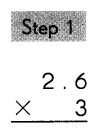Multiply the tenths by 3.
3 × 6 tenths = _18_ tenths
Regroup the tenths.
18___ tenths = _1__ one and __6_ tenths
Step 2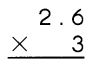Multiply the tenths by 3.
3 × 2 ones = _6__ ones
_6__ ones + _1_ one = _7_ ones
So, 3 × 2.6 = _7.8__

Multiply.

Question 8.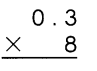Explanation:
Multiply the tenths by 8.
8 × 3 tenths = 24 tenths
Regroup the tenths.
24 tenths = 2 one and  4 tenths
Step 2Multiply the tenths by 8.
8 × 0 ones = 0 ones
0 ones + 2 one = 2 ones
So, 0.3 × 8 = 2.4

Question 9.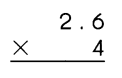Explanation:
Multiply the tenths by 4.
4 × 6 tenths = 24 tenths
Regroup the tenths.
24 tenths = 2 one and  4 tenths
Step 2Multiply the tenths by 4.
4 × 2 ones = 8 ones
8 ones + 2 one = 10 ones
So, 2.6 × 4 = 10.4

Question 10.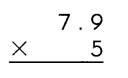Explanation:
Multiply the tenths by 5.
5 × 9 tenths = 45 tenths
Regroup the tenths.
45 tenths = 4 one and  5 tenths
Step 2Multiply the tenths by 5.
5 × 7 ones = 35 ones
35 ones + 4 one = 39 ones
So, 7.9 × 5 = 39.5

Question 11.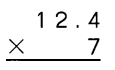Explanation:
Multiply the tenths by 7.
7 × 4 tenths = 28 tenths
Regroup the tenths.
28 tenths = 2 one and  8 tenths
Step 2Multiply the tenths by 5.
7 × 12 ones = 84 ones
84 ones + 2 one = 86 ones
So, 12.4 × 7 = 86.8

Question 12.
Step 1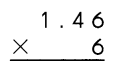Explanation:
Multiply the hundredths by 6.
6 × 6 hundredths =36 hundredths
Regroup the tenths.
36 hundredths = 3 tenths and 6 hundredths

Step 2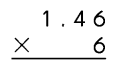Multiply the tenths by 6.
6 × 4 tenths = 24 tenths
24 tenths + 3 tenths = 27 tenths
Regroup the tenths.
27 tenths = 2 ones and 7 tenths

Step 3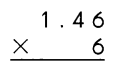Multiply the ones by 6.
6 × 1 ones = 6 ones
6 ones + 2 ones = 8 ones
So, 6 × 1.46 = 8.76

Multiply.

Question 13.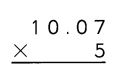Explanation:
Multiply the hundredths by 5.
5 × 7 hundredths =35 hundredths
Regroup the tenths.
35 hundredths = 3 tenths and 5 hundredths

Step 2Multiply the tenths by 5.
5 × 0 tenths = 0 tenths
0 tenths + 3 tenths = 3 tenths
Regroup the tenths.
3 tenths = 0 ones and 3 tenths

Step 3Multiply the ones by 6.
5 × 10 ones = 50 ones
50 ones + 0 ones = 50 ones
So, 5 × 10.07 = 50.35

Question 14.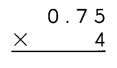Explanation:
Multiply the tenths by 4.
4 × 5 tenths = 20 tenths
Regroup the tenths.
20 tenths = 2 one and  0 tenths
Step 2Multiply the tenths by 4.
4 × 7 ones = 28 ones
28 ones + 2 one = 2 ones
So, 0.75 × 4 = 3

Question 15.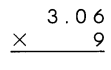Explanation:
Multiply the hundredths by 9.
9 × 6 hundredths =54 hundredths
Regroup the tenths.
54 hundredths = 5 tenths and 4 hundredths

Step 2Multiply the tenths by 9.
9 × 0 tenths = 0 tenths
0 tenths + 54 tenths = 54 tenths
Regroup the tenths.
54 tenths = 5 ones and 4 tenths

Step 3Multiply the ones by 9.
9 × 3 ones = 27 ones
27 ones + 0 ones = 27 ones
So, 9 × 3.06 = 27.54

Question 16.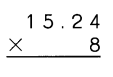Explanation:
Multiply the hundredths by 8.
8 × 4 hundredths =32 hundredths
Regroup the tenths.
32 hundredths = 3 tenths and 2 hundredths

Step 2Multiply the tenths by 8.
8 × 2 tenths = 16 tenths
16 tenths + 3 tenths = 3 tenths
Regroup the tenths = 19

Step 3Multiply the ones by 8.
8 × 15 ones = 120 ones
120 ones + 1 ones = 121 ones
So, 8 × 15.24 = 121.92

Question 17.
4 × 2.08 = ___
Explanation:
Multiply the hundredths by 4.
4 × 8 hundredths =32 hundredths
Regroup the tenths.
32 hundredths = 3 tenths and 2 hundredths

Step 2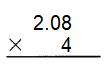Multiply the tenths by 4.
4 × 0 tenths = 16 tenths
0 tenths + 3 tenths = 3 tenths

Step 3Multiply the ones by 4.
4 × 2 ones = 8 ones
8 ones + 32 ones = 8.32 ones
So, 4 × 2.o8 = 8.32

Question 18.
3 × 3.29 = ___
Explanation:
Multiply the hundredths by 3.
9 × 3 hundredths =27 hundredths
Regroup the tenths.
27 hundredths = 2 tenths and 7 hundredths

Step 2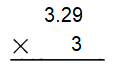Multiply the tenths by 3.
3 × 2 tenths = 6 tenths
6 tenths + 0 tenths = 6 tenths
Regroup the tenths
6 tenths + 2 tenths = 8 tenths

Step 3Multiply the ones by 6.
6 × 3 ones = 18 ones
8 ones + 1 ones = 9 ones
So, 3 × 3.29 = 9.87

Question 19.
7 × 5.71 = ___
Explanation:
Multiply the hundredths by 7.
1 × 7 hundredths =7 hundredths
Regroup the tenths.
7 hundredths = 0 tenths and 7 hundredths

Step 2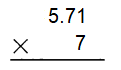Multiply the tenths by 7.
7 × 7 tenths = 49 tenths
49 tenths + 0 tenths = 49 tenths
Regroup the tenths
49 tenths = 4 ones and 7 tenths

Step 3Multiply the ones by 7.
5 × 7 ones = 35 ones
35 ones + 4 ones = 39 ones
So, 7 × 5.71 = 39.97

Question 20.
6 × 4.81 = ___
Explanation:
Multiply the hundredths by 6.
1 × 6 hundredths =6 hundredths
Regroup the tenths.
6 hundredths = 0 tenths and 6 hundredths

Step 2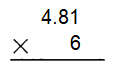Multiply the tenths by 6.
8 × 7 tenths = 48 tenths
48 tenths + 0 tenths = 48 tenths
Regroup the tenths
48 tenths = 4 ones and 8 tenths

Step 3Multiply the ones by 6.
4 × 6 ones = 24 ones
24 ones + 4 ones = 28 ones
So, 6 × 4.81 = 28.81

Question 21.
9 × 7.46 = ___
Explanation:
Multiply the hundredths by 9.
9 × 6 hundredths =54 hundredths
Regroup the tenths.
54 hundredths = 5 tenths and 4 hundredths

Step 2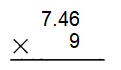Multiply the tenths by 9.
9 × 4 tenths = 36 tenths
36 tenths + 5 tenths = 41 tenths
Regroup the tenths
41 tenths = 4 ones and 1 tenths

Step 3Multiply the ones by 9.
9 × 7 ones = 63 ones
63 ones + 4 ones = 67 ones
So, 9 × 7.46 = 67.14

Question 22.
8 × 6.52 = ___
Explanation:
Multiply the hundredths by 8.
8 × 2 hundredths =16 hundredths
Regroup the tenths.
16 hundredths = 1 tenths and 6 hundredths

Step 2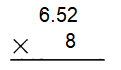Multiply the tenths by 8.
8 × 5 tenths = 40 tenths
40 tenths + 1 tenths = 41 tenths
Regroup the tenths
41 tenths = 4 ones and 1 tenths

Step 3Multiply the ones by 8.
8 × 6 ones = 48 ones
48 ones + 4 ones = 52 ones
8 × 6.52 = 52.16

Write the correct decimal in each box.

Example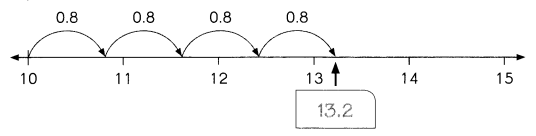Question 23.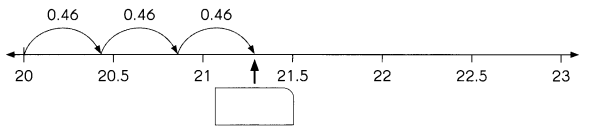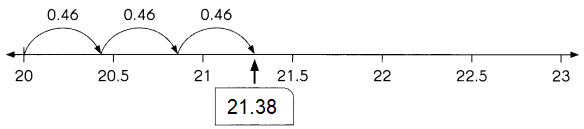Explanation:
0.46 x 3
3 × 0.46 = 3 × 46 hundredths
= 138 hundredths
= 1.38
So, 3 × 0.46 = 1.38
20+1.38 = 21.38

Question 24.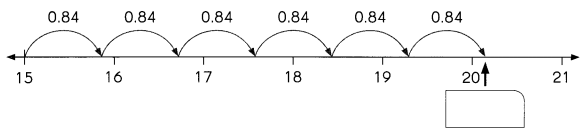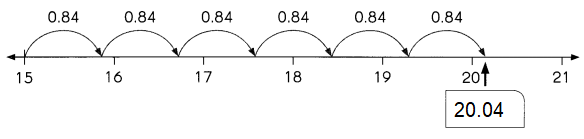Explanation:
6 x 0.84
6 × 0.84 = 6 × 84 hundredths
= 504 hundredths
= 5.04
So, 6 × 0.84 = 5.04
15+5.04 = 20.04

Question 25.